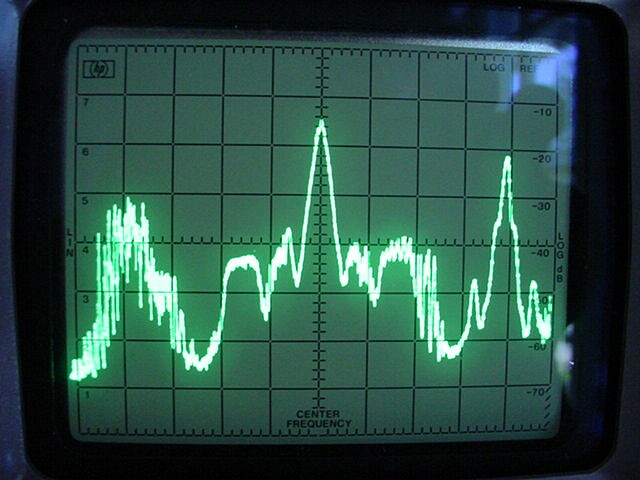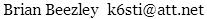Nearly all stereo decoders use a 38 kHz square wave to demodulate the L−R signal, which occupies 23 to 53 kHz in the stereo composite. An unintended consequence is that the waveform's fifth harmonic demodulates power near 190 kHz. HD Radio digital sidebands, which appear at 129 to 198 kHz for hybrid service mode MP1, can cause an annoying background noise when the fifth harmonic demodulates them. Extended hybrid signals, whose spectrum goes down to 115.5 kHz for service mode MP3, cause additional noise due to the third harmonic at 114 kHz. Furthermore, FM detection can generate intermodulation products between the digital sidebands and the analog modulation. The analysis below does not address this issue, but Dave Hershberger analyzes it here.

A combination of measurement and calculation yields the analog signal-to-noise ratio (S/N) for HD Radio self-noise due to a squarewave stereo decoder. I make separate assessments for service mode MP1 and extended hybrid modes MP2, MP3, and MP11 that provide additional channel capacity.This is the RF spectrum of KUSC in Los Angeles on an HP 141T/8553B/8552B spectrum analyzer. The horizontal scale is 100 kHz/div and vertical is 10 dB/div. At the center of the screen is the analog carrier at 91.5 MHz. 50 to 100 kHz away are RDS and SCA subcarriers. 130 to 200 kHz out are the digital sidebands. A weak station at 91.7 mixes with KUSC's upper digital sideband. To the right is a station at 91.9 with SCA.This is the detected spectrum in a Yamaha T-1020 FM tuner, which uses two 250 kHz ceramic IF filters, a quadrature detector, and no postdetection filter. The frequency span is 0 to 200 kHz and the analysis filter bandwidth is 3 kHz. The leftmost pip is DC. The next pip is the 19 kHz pilot signal. Then comes a low-level RDS subcarrier at 57 kHz, followed by SCA subcarriers at 67 kHz and 92 kHz. At about 130 kHz the digital signal begins. It extends to about 200 kHz.

I adjusted the trace so that the pilot was about 20 dB down. Later I learned from the station engineer that the pilot was set at 8.5% deviation, the SCAs at 9.2% each, and RDS at 1.3%. Total subcarrier deviation was 19.7%. If peak carrier deviation was increased beyond 100% by 0.5% for each 1% of subcarrier deviation as permitted by FCC rules, it would be 109.85%. This leaves 81.65% for the program channel, putting it 19.7 dB above the pilot, or 0.3 dB below the top of the screen. For S/N reference I use a 100%-deviated, 1 kHz sine wave. The receiver's 75 µs deemphasis network attenuates this signal 0.9 dB so the effective reference level is 0.3 + 0.9 = 1.2 dB below the top of the screen. Call this 0 dBr.

To minimize round-off errors, I'll carry figures in tenths of a dB even though off-screen measurements are not nearly that accurate. The detected noise near 190 kHz is about 38 dB below full scale, or −36.8 dBr. The HP 8552B IF section uses average-log instead of RMS-linear detection, which results in a 2.5 dB undermeasurement for noise. In addition, the equivalent noise bandwidth of the analysis filter is slightly greater than its −3 dB bandwidth, resulting in a 0.5 dB overmeasurement. The detected noise level near 190 kHz therefore is −36.8 dBr + 2.5 − 0.5 = −34.8 dBr.

The digital sidebands are each 23 dB below the analog carrier, or −20 dBc total. This figure should be accurate for this signal since KUSC uses a single antenna for both analog and digital. The sidebands are 69 kHz wide so the measured level in a 3 kHz equivalent noise bandwidth will be 10log(69 ⁄ 3) = 13.6 dB lower, or −33.6 dBc. This level corresponds to −34.8 dBr. Therefore, dBr = dBc − 1.2. This relation is valid for this tuner and signal near 190 kHz.

A stereo decoder with a 38 kHz squarewave oscillator will demodulate noise near 190 kHz with the fifth harmonic. The 75 µs deemphasis network rolls off the audio. The digital sidebands are composed of OFDM subcarriers at −45.8 dBc spaced 363.373 Hz apart. I used these facts in a simple computer program to calculate the total deemphasized noise contributed by the subcarriers between 175 kHz, which demodulates to 15 kHz audio, and the last one at 198.402 kHz. The calculation yields −33.7 dBc.

A square wave's fifth harmonic is 14.0 dB below the fundamental, so the noise level becomes −33.7 dBc − 14.0 − 1.2 = −48.9 dBr after stereo decoding and deemphasis. In other words, S/N for the KUSC analog signal is 48.9 dB for service mode MP1.

How much additional noise does service mode MP2 bring? In this mode the digital sidebands extend down to 122.457 kHz. The third harmonic of 38 kHz at 114 kHz will demodulate the noise between this frequency and 129 kHz, which corresponds to 15 kHz audio, as −47.6 dBc. A square wave's third harmonic is 9.5 dB below the fundamental. Therefore the demodulated and deemphasized noise level is −47.6 dBc − 9.5 − 1.2 = −58.3 dBr. This figure must be adjusted for the difference in IF-filter response between 190 kHz, where the 1.2 dB relation was derived, and the MP2 region centered at 126 kHz.

To determine the response difference, I fed two signal generators through a power combiner to the T-1020. I set the tuner and one generator, modulated with a 9% pilot, at 98.500 MHz. I set the other generator at 98.690 MHz and adjusted its RF level until the detected signal at 190 kHz was about 20 dB below the pilot. Then I set the spectrum analyzer to 2 dB/div and measured the level change when I moved the generator to 98.626 MHz. I repeated the test on the lower side of the carrier and averaged the two readings. The result was 4.5 dB. This is the change in tuner response between 190 and 126 kHz offsets for a low-level, additive RF signal.

The adjusted MP2 result is −58.3 dBr + 4.5 = −53.8 dBr. Summing the −48.9 dBr noise from the fifth harmonic yields −47.7 dBr total.

Service mode MP3 extends the digital sidebands down to 115.553 kHz. Noise from this frequency to 129 kHz calculates as −38.8 dBc, which becomes −49.5 dBr when demodulated. IF response at 122 kHz was 4.6 dB higher than at 190 kHz, yielding −44.9 dBr. Summing the −48.9 dBr noise from the fifth harmonic gives −43.4 dBr total.

Service mode MP11 extends the digital sidebands down to 101.744 kHz. Noise to 129 kHz calculates as −33.6 dBc, and demodulated noise as −39.7 dBr, including 4.6 dB greater IF response at 115 kHz. Total noise from the third and fifth harmonics is −39.2 dBr.

These calculations are based on a signal with two SCAs, RDS, 8.5% pilot, and 81.65% program modulation. For a more typical signal with no SCAs, no RDS, 9% pilot, and 91% program modulation, S/N increases by 20log(0.91/0.8165) = 0.94 dB. For the table below I've added 0.9 dB to the S/N figures calculated above, listed the S/N differences from MP1 for the other service modes at 0.1 dB resolution, and rounded the absolute S/N values to the nearest dB.

```   Mode    Analog S/N  Difference
MP1       50 dB     reference
MP2       49 dB      -1.2 dB
MP3       44 dB      -5.5 dB
MP11      40 dB      -9.7 dB
```

The calculated figures depend on rather imprecise off-screen level measurements for a single station, tuner, and RF signal level. Even with these limitations, the figures should be accurate to within a few dB for any tuner with two wideband ceramic IF filters, no postdetection filter, and a squarewave stereo decoder. This covers the great majority of older home tuners and receivers. Narrower IF filters help a lot. I've seen a worthwhile reduction in MP1 self-noise when replacing 280 kHz filters with 230s, and a substantial reduction with 180s. A postdetection filter or 38 kHz harmonic canceller can greatly reduce or eliminate HD Radio self-noise.

The S/N figures are for digital sidebands at −20 dBc. Adjust the result for higher sideband levels. For example, for −14 dBc sidebands subtract 6 dB from the S/N values. Note that intermodulation products in the composite passband described in the Hershberger article increase twice as fast as the digital sideband level increases.

The S/N figures reference a 100%-deviated, 1 kHz sine wave. RMS levels for minimally processed program material with wide dynamic range may be 25 dB lower at times. Stations with conservative audio processing can expect MP1 self-noise sometimes to be just 50 − 25 = 25 dB below the program level and distinctly audible.

## Noise Calculation

```	DEFINT I				' Integer variable
CONST fc = 1 / (2 * 3.141593 * 75E-6)	' 75 us deemphasis corner freq
CONST s = 1488375 / 4096		' OFDM subcarrier spacing in Hz
DECLARE FUNCTION db (p)			' dB from power
FOR i = 175000 / s TO 546		' 15 kHz below 5th harmonic to last subcarrier
f = i * s - 190000			' Decoded freq
a = a + 1 / (1 + (f / fc) ^ 2)	' Sum deemphasized subcarrier powers
NEXT i
FOR i = 337 TO 129000 / s		' First MP2 subcarrier to 15 kHz above 3rd harmonic
f = i * s - 114000
b = b + 1 / (1 + (f / fc) ^ 2)
NEXT i
FOR i = 318 TO 129000 / s		' First MP3 subcarrier to 15 kHz above 3rd harmonic
f = i * s - 114000
c = c + 1 / (1 + (f / fc) ^ 2)
NEXT i
FOR i = 280 TO 129000 / s		' First MP11 subcarrier to 15 kHz above 3rd harmonic
f = i * s - 114000
d = d + 1 / (1 + (f / fc) ^ 2)
NEXT i
PRINT USING "###.# "; db(a); db(b); db(c); db(d)
FUNCTION db(p)				' Power ratio -> dB for -20 dBc sidebands
db = 10 * LOG(p) / LOG(10) - 45.8	' LOG is natural log
END FUNCTION
```

## CRC Measurements

Communications Research Centre Canada published a report on HD Radio compatibility. Beginning on page 24, it gives self-noise levels for 14 recent but unidentified FM receivers for MP1, MP3, and MP11 service modes at −20, −14, and −10 dBc. Although the modulation and S/N standards differ from those used here, the figures illustrate the effect of service mode and sideband level as well as the variation among contemporary receivers.

October 19, 202288–108 MHz